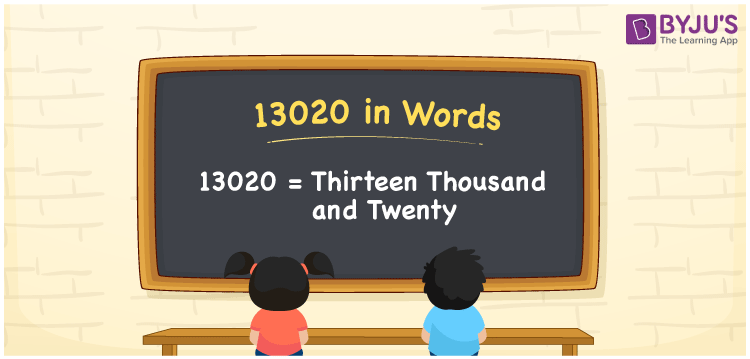# 13020 in words

13020 in words is written as Thirteen Thousand and Twenty. In 13020, 1 has a place value of ten thousand, 3 is in the place value of thousand and 2 is in the place value of ten. The article on Place Value gives more information. The number 13020 is used in expressions that relate to money, distance, length, Social media views, area and many more. For example, “The car parking space in an apartment was ThirteenThousand and Twenty meters long”.

 13020 in words Thirteen Thousand and Twenty Thirteen Thousand and Twenty in Numerals 13020

## 13020 in English Words## How to Write 13020 in Words?

We can convert 13020 to words using a place value chart. The number 13020 has 5 digits, so let’s make a chart that shows the place value up to 5 digits.

 Ten thousand Thousands Hundreds Tens Ones 1 3 0 2 0

Thus, we can write the expanded form as:

1 × Ten thousand + 3 × Thousand + 0 × Hundred + 2 × Ten + 0 × One

= 1 × 10000 + 3 × 1000 + 0 × 100 + 2 × 10 + 0 × 1

= 13020.

= Thirteen Thousand and Twenty.

13020 is the natural number that is succeeded by 13019 and preceded by 13021.

13020 in words – Thirteen Thousand and Twenty.

Is 13020 an odd number? – No.

Is 13020 an even number? – Yes.

Is 13020 a perfect square number? – No.

Is 13020 a perfect cube number? – No.

Is 13020 a prime number? – No.

Is 13020 a composite number? – Yes.

## Solved Example

Question:

Write the number 13020 in expanded form

Solution:

13020 = 1 × Ten Thousand + 3 × Thousands  + 0 × Hundreds + 2 × Tens + 0 × One

13020 =  1 × 10000 + 3 × 1000 + 0 × 100 + 2 × 10 + 0 × 1

13020 =  10000 + 3000 + 0 + 20 + 0

13020 =  10000 + 3000 + 20

## Frequently Asked Questions on 13020 in words

Q1

### How to write the number 13020 in words?

13020 in words is written as Thirteen Thousand and Twenty.
Q2

### State whether True or False. 13020 is divisible by 3?

True. 13020 is divisible by 3.
Q3

### Is 13020 divisible by 10?

Yes. 13020 is divisible by 10.
Test your Knowledge on 13020 in Words